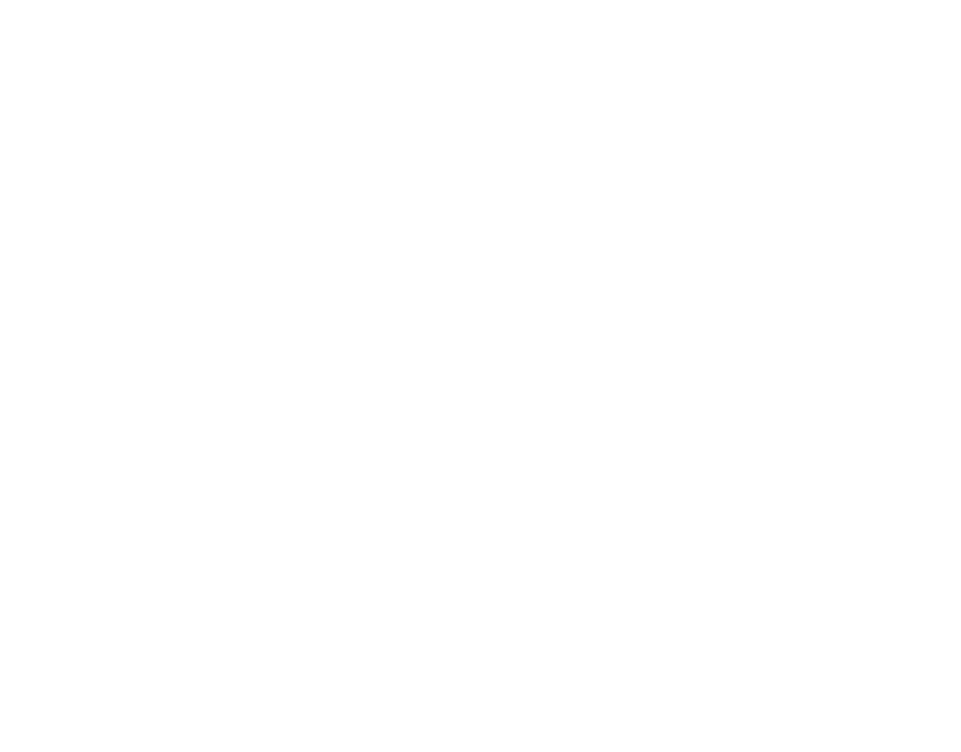# Variable and Fixed Costs

Variable Costs and Fixed Costs are a type of classification of costs based on their behavior pattern in relation to volume or activity of the business. In short, total variable cost varies in proportion to the change in output / activity / volume of the business whereas the total fixed costs remain the same. Based on their behavior with respect to the change in output, their names are variable and fixed.

## Variable Cost

As hinted earlier, variable costs are those costs which are output dependent. Most common example of variable costs is direct material cost. When there is a positive correlation between the production output and the total cost of a particular material, such material cost should be categorized as the variable cost. We should note that total variable cost varies with output but per unit variable cost remains fixed. These costs are easily and automatically controllable because they are incurred when required.

## Fixed Cost

Unlike, variable costs, fixed costs are not output dependent. The best example of fixed costs is the machinery cost, supervision cost, etc. Generally, the fixed costs are either incurred in advance or they are almost certain to incur irrespective of the activity levels of the manufacturing unit. Therefore, it is difficult to control fixed costs in a short span of time. Controlling fixed costs requires special attention of the manager. The notable point here is that in the very long term, all costs are variable costs. Fixed costs are fixed till a certain level of activity. Total fixed costs remain unchanged but per unit fixed costs changes with output level.### How to identify a cost as Variable or Fixed?

At the very outset, we should understand that same cost can be variable or fixed under different circumstances. Suppose a machinery is required to be replaced every 2 years. The cost of machinery is fixed cost if we look at a term of say 1 year but if we take a term of 10 years, it is also a variable cost. Now, suppose a machinery is required to be replaced after every 1 million units of production. The cost of machinery is fixed if we consider 10,000 units, but if we consider, 50 million units, a cost of machinery is a variable cost. In essence, there are two things which differentiate a cost between fixed or variable i.e. Given Time Period and Specific Activity Level.

### Benefit of classification between variable and fixed costs

With the help of this classification only, a manager can find out its breakeven point which acts as a planning guide for him. The ratio of variable cost to fixed costs shows how much adjustable an organization is to the changing situations. Higher the percentage of variable costs, lower is the chances of that organization going into losses. Higher fixed costs have complications related to under-utilization etc. Slowly and gradually a manager may think of making most of its costs variable if he perceives bad economic conditions. Fixed costs give a leverage when the volume of sales is high but when that is lower than the breakeven point, same fixed costs take organizations towards losses.

Share Knowledge if you liked1.